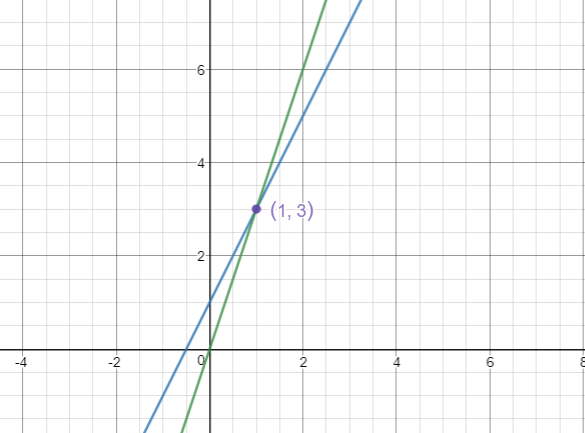# Solving Simultaneous Equations

## Finding a solution to two linear equations

Simultaneous equations are two equations that at meet at one particular point ( if they are linear, often more if not!). For linear equations this will involve an x and a y value which is the same for both equations. Here are the steps required to solve two linear simultaneous equations:

Step 1: Write out the equations and label them

__A __3x+7y=23

B 2x +3y=12

In order to solve the equations we need to eliminate one variable. To do this we need to make one variable equal.

Step 2: Multiply the equations to make at least one variable equal

_A __ __3__x+7y=23 x2 _You can choose to make the x or y equal, I am going to choose x

__B __ __2__x +3y=12 x3

I am going to make a common multiple of x in equation A by multiplying it all by 2.

I am going to make a common multiple of x in equation B by multiplying it all by 3.

A __3__x+7y=23 x2 = __6__x+14y=46

B __2__x +3y=12 x3 = __6__x +9y=36 Now we have a common coefficient of x

Step 3: I now need to get rid of the x variable. Because I have positive two positive 6x I am going to subtract A from B.

__A __ = __6__x+14y=46

__B __ = __6__x +9y=36 / 0 +5y=10

Step 4: Solve for one variable. Now I have one unknown I can work out y:

5y=10

y=2

Step 5: Substitute you value into an original equation. (It doesn’t matter which one!)

Substitute in y=2

__A __3x+7y=23

3x+7(2)=23

3x+14=23

3x=9

x=3

Step 6: Check by substituting in both values into the other original formula

y=2 x=3

__B __ 2x +3y=12

2(3)+3(2)= 12

6+6=12 Therefore we are correct!

Take care with Step 3, for example sometimes you might have two equations that you need to add. For example, if you get two equations such as:

3x +6y =12

2x - 6y= 9

To cancel out the +6y with the -6y you will need to add as 6y +-6y =0

So this would simplify down to 5x=21

You would then solve from this point.

## Finding solutions to two linear equations graphically

To solve two linear simultaneous equations graphically you simply need to draw the graphs and look for the point where the two lines intersect.Sometimes this may involve rearranging equations to put them into the format y=mx+c which will make it easier for you to draw the graphs.

Solve the simultaneous equation 4x + 3y = 6 and 3x + y = 7